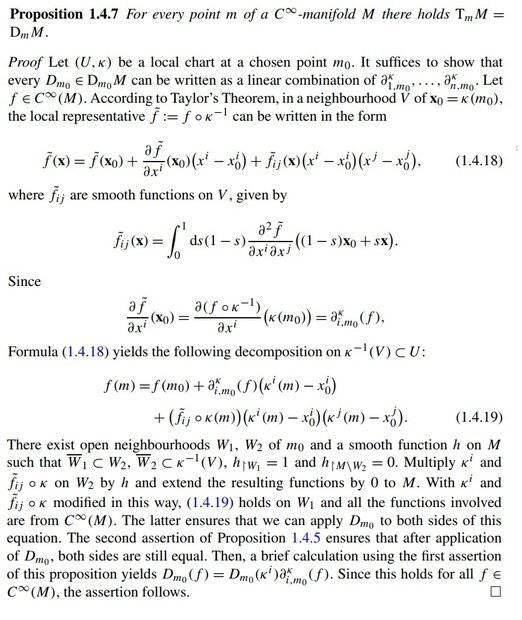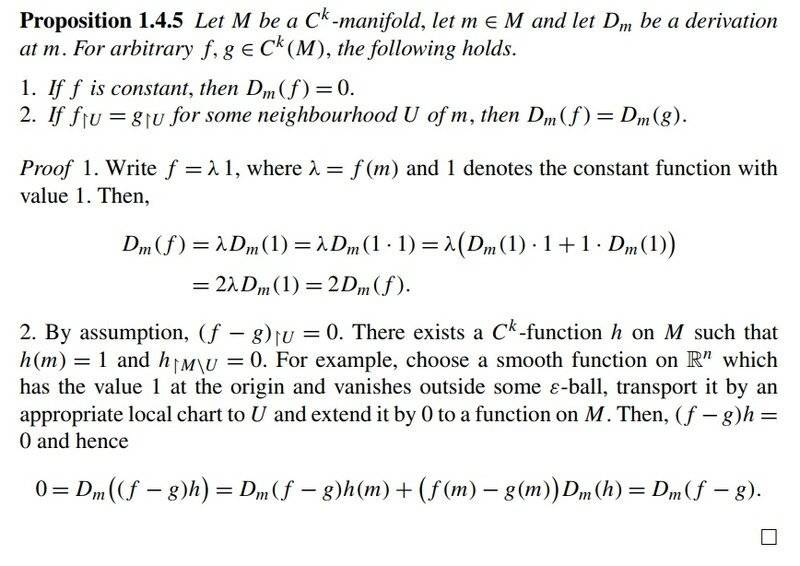# Derivations and Derivatives

• I
• Korybut

#### Korybut

TL;DR Summary
Need some clarifying commentary on the proof
Hello!
According to the attached proposition on ##C^\infty## manifold space of derivations ##D_m M## is isomorphic to Tangent space ##T_m M##.Cited here another proposition (1.4.5) states the following
1. For constant function ##D_m(f)=0##
2. If ##f\vert_U=g\vert_U## for some neighborhood ##U## of ##m##, then ##D_m(f)=D_m(g)##.

I don't get the following.
1. Where ##C^\infty## is important? I think that this is important due to algebraic reasons. In Teylor's formula all terms should of the same ##C^k## and this happens only for ##C^\infty##. Am I right?
2. I don't get this ##h##-thing completely. Even without it I can apply derivation ##D_{m_0}## to both sides of (1.4.19) and get statement of the proposition. What am I missing?

Summary:: Need some clarifying commentary on the proof

Hello!
According to the attached proposition on ##C^\infty## manifold space of derivations ##D_m M## is isomorphic to Tangent space ##T_m M##.
View attachment 296438
Cited here another proposition (1.4.5) states the following
1. For constant function ##D_m(f)=0##
2. If ##f\vert_U=g\vert_U## for some neighborhood ##U## of ##m##, then ##D_m(f)=D_m(g)##.

I don't get the following.
1. Where ##C^\infty## is important?
This is simply because it is easier to bother with smooth functions than to administrate an additional parameter in a proof that is very technical anyway. What are the assumptions in 1.4.5?

I think that this is important due to algebraic reasons. In Teylor's formula all terms should of the same ##C^k## and this happens only for ##C^\infty##. Am I right?
2. I don't get this ##h##-thing completely. Even without it I can apply derivation ##D_{m_0}## to both sides of (1.4.19) and get statement of the proposition. What am I missing?
I guess that your quotation of 1.4.5 is incomplete. What do we have outside of ##U##? How can ##f\vert_U=g\vert_U## determine the behaviour of ##D_m(f)## outside of ##U##? Either you have an additional property of ##M##, e.g. a transitive operation, or ##f\vert_{M/U}=g\vert_{M/U}=0.## The latter would explain the role of ##h.##

•Korybut
This is simply because it is easier to bother with smooth functions than to administrate an additional parameter in a proof that is very technical anyway. What are the assumptions in 1.4.5?
Do you mean that one can prove this isomorphism ##T_m M=D_m M## without assuming that manifold is of ##C^\infty##?
This is simply because it is easier to bother with smooth functions than to administrate an additional parameter in a proof that is very technical anyway. What are the assumptions in 1.4.5?

I guess that your quotation of 1.4.5 is incomplete. What do we have outside of ##U##? How can ##f\vert_U=g\vert_U## determine the behaviour of ##D_m(f)## outside of ##U##? Either you have an additional property of ##M##, e.g. a transitive operation, or ##f\vert_{M/U}=g\vert_{M/U}=0.## The latter would explain the role of ##h.##
Indeed. Sorry for that here is the full proposition with the proofProof of this proposition also uses this ##h##-thing. I used to think that it was made just to make the proof look fancier. Now I believe that I miss something very subtle...

It looks it is very important for derivations to act only on functions that are defined globally on whole manifold. (1.4.19) holds only in some neighbourhood. However while l.h.s. is well-defined for entire manifold, on the other hand r.h.s. is defined only in this neighbourhood and with this ##h##-trick acquires definition on the entire manifold.
Why it is so important for derivation to be applied only on globally defined function? I know that according to the definition ##D_m\, :\, C^k(M)\rightarrow \mathbb{R}##. But this is derivation a point which looks like very local operation... Ahhhh

Do you mean that one can prove this isomorphism ##T_m M=D_m M## without assuming that manifold is of ##C^\infty##?
All I can see is, that we need an integrable second derivative. The theorem is probably meant to use for Lie algebras, i.e. Lie groups, i.e. analytic manifolds, i.e. smooth manifolds. Derivations are another point of view for the Leibniz rule or the Jacobi identity, or: how to differentiate a product?

Smooth manifolds are a lot more convenient than tangential spaces on ##C^k(M)## and the most interesting examples are smooth manifolds.

Indeed. Sorry for that here is the full proposition with the proof0View attachment 296443
Proof of this proposition also uses this ##h##-thing. I used to think that it was made just to make the proof look fancier. Now I believe that I miss something very subtle...

It looks it is very important for derivations to act only on functions that are defined globally on whole manifold. (1.4.19) holds only in some neighbourhood. However while l.h.s. is well-defined for entire manifold, on the other hand r.h.s. is defined only in this neighbourhood and with this ##h##-trick acquires definition on the entire manifold.
Why it is so important for derivation to be applied only on globally defined function? I know that according to the definition ##D_m\, :\, C^k(M)\rightarrow \mathbb{R}##. But this is derivation a point which looks like very local operation... Ahhhh
The ##h-##thingy as you call it is the partition of the unity. It bridges the gap between local and global. Differentiation is a local property, but we want to make a statement about the global manifold, and that its tangents at ##m\in M## are derivatives in ##m## for any ##m.## Remember that the derivative of a one-dimensional function is a linear function that obeys the Leibniz rule. Proposition 1.4.7 is simply the multi-dimensional version. In order to get a global statement from a local property, it is useful to expand the local function by zero outside the local neighborhood. For example, the normal field of a submanifold is to be extended to the entire manifold. Or: In order to define the area integral, or to integrate over manifolds in general, coordinates must be chosen, which is only possible locally. The integrand must therefore be broken down in such a way that it remains locally integrable, but becomes zero outside the scope of the coordinate system. That is what ##h## is for. It is the analytical version of Urysohn's Lemma.

•Korybut
All I can see is, that we need an integrable second derivative. The theorem is probably meant to use for Lie algebras, i.e. Lie groups, i.e. analytic manifolds, i.e. smooth manifolds. Derivations are another point of view for the Leibniz rule or the Jacobi identity, or: how to differentiate a product?

Smooth manifolds are a lot more convenient than tangential spaces on Ck(M) and the most interesting examples are smooth manifolds.
I do understand what you are talking about. But I believe the reason is simply formal. If ##f## is of class ##C^k(M)##. Then l.h.s. of (1.4.19) is ##C^k## and I can apply derivation that maps ##C^k## functions to real numbers. However the remainder in Teylor's formula is of class ##C^{k-2}## and it is not clear how one should apply the same derivation to this term.

One more time. Thanks a lot for your help!

Last edited:
Why it is so important for derivation to be applied only on globally defined function?
I am guessing that is how the definitions were given in the book (which one is it?). Otherwise you get something equivalent. The space of derivations at a point of ##C^\infty(M)## and the space of derivations at a point of the algebra of locally defined smooth functions around the point are isomorphic. But they have to be consistent with their definitions.

I am guessing that is how the definitions were given in the book (which one is it?). Otherwise you get something equivalent. The space of derivations at a point of ##C^\infty(M)## and the space of derivations at a point of the algebra of locally defined smooth functions around the point are isomorphic. But they have to be consistent with their definitions.
I also believe that all this machinery is because of this very specific definition that derivation works only on function that are defined globally on the whole manifold. Book is "Differential Geometry and Mathematical Physics" by Rudolph and Schmidt (very hard to read (for me) but rigorous).

I also believe that all this machinery is because of this very specific definition that derivation works only on function that are defined globally on the whole manifold. Book is "Differential Geometry and Mathematical Physics" by Rudolph and Schmidt (very hard to read (for me) but rigorous).
Yes, definition 1.4.4 page 24.

•Korybut